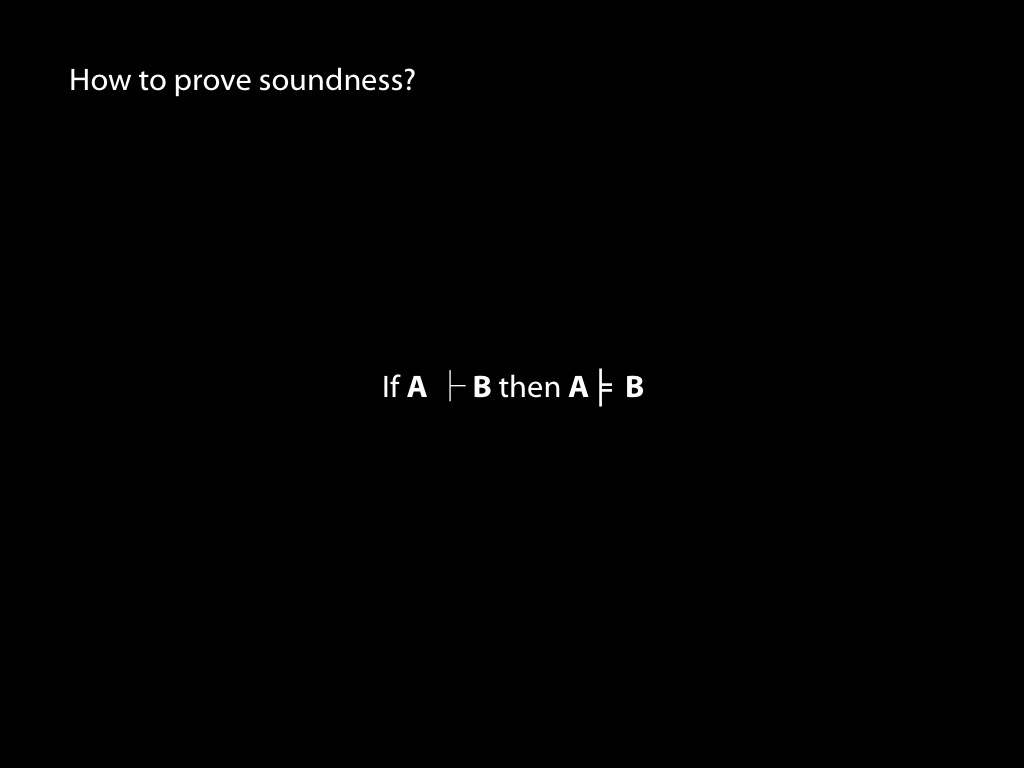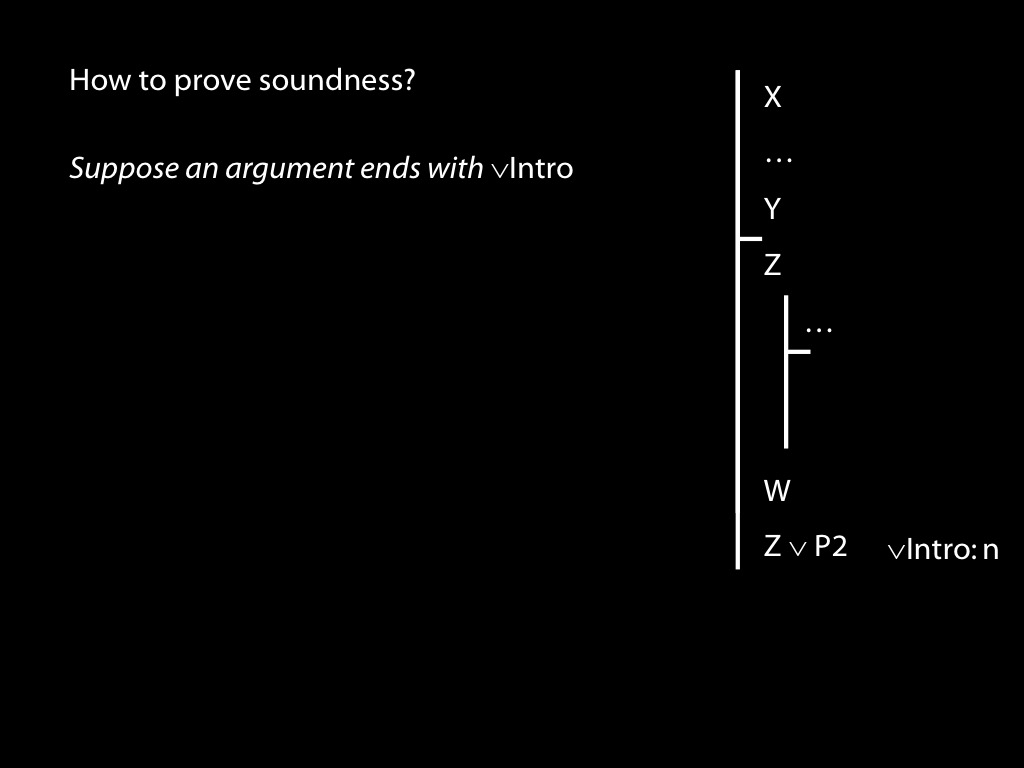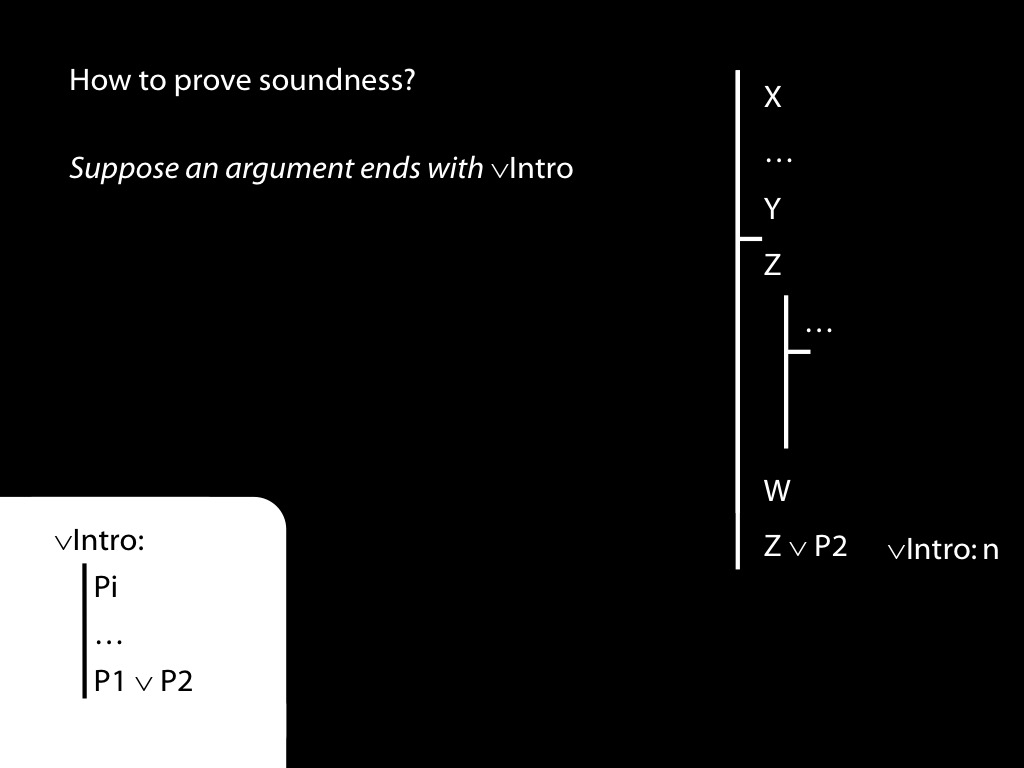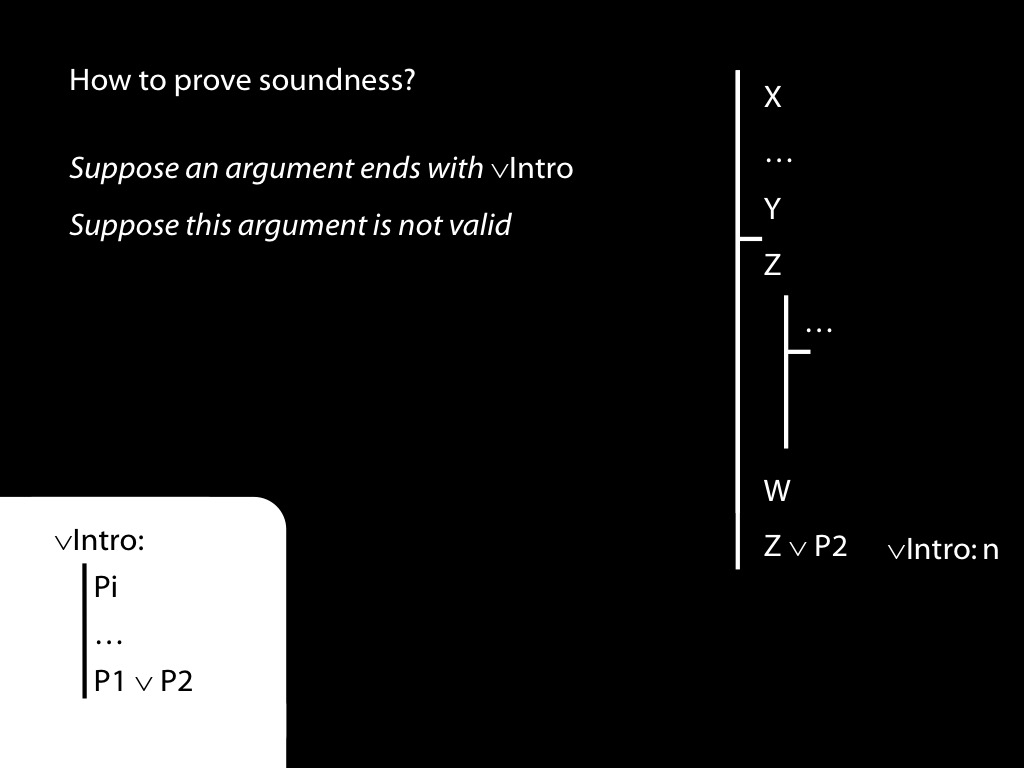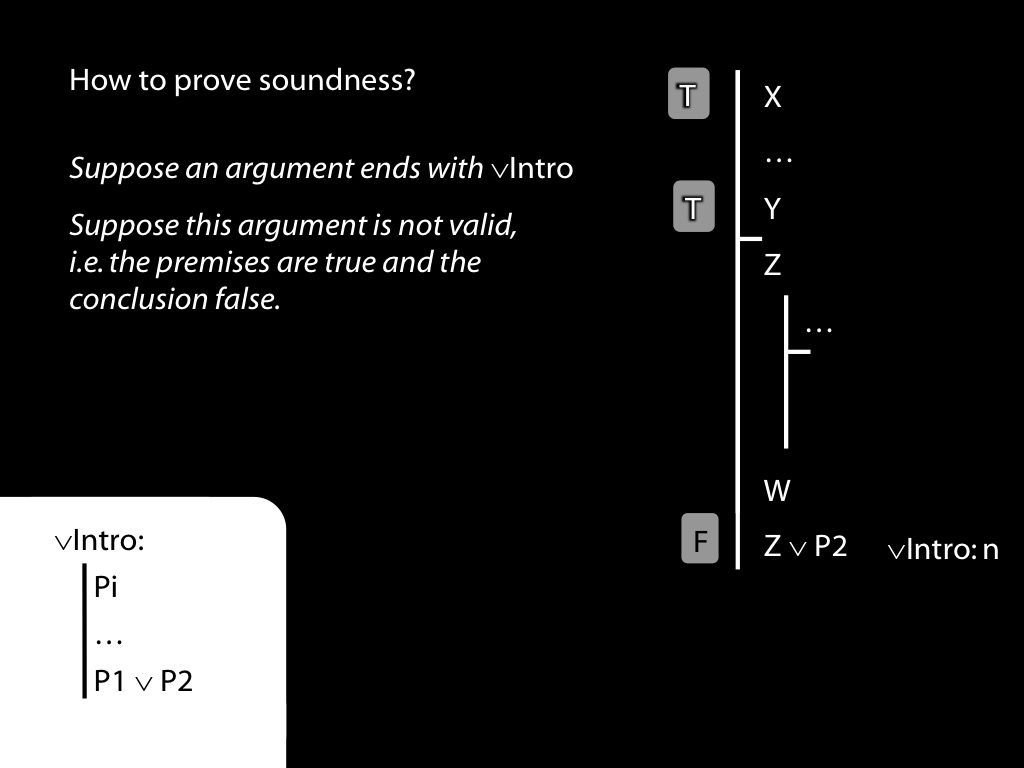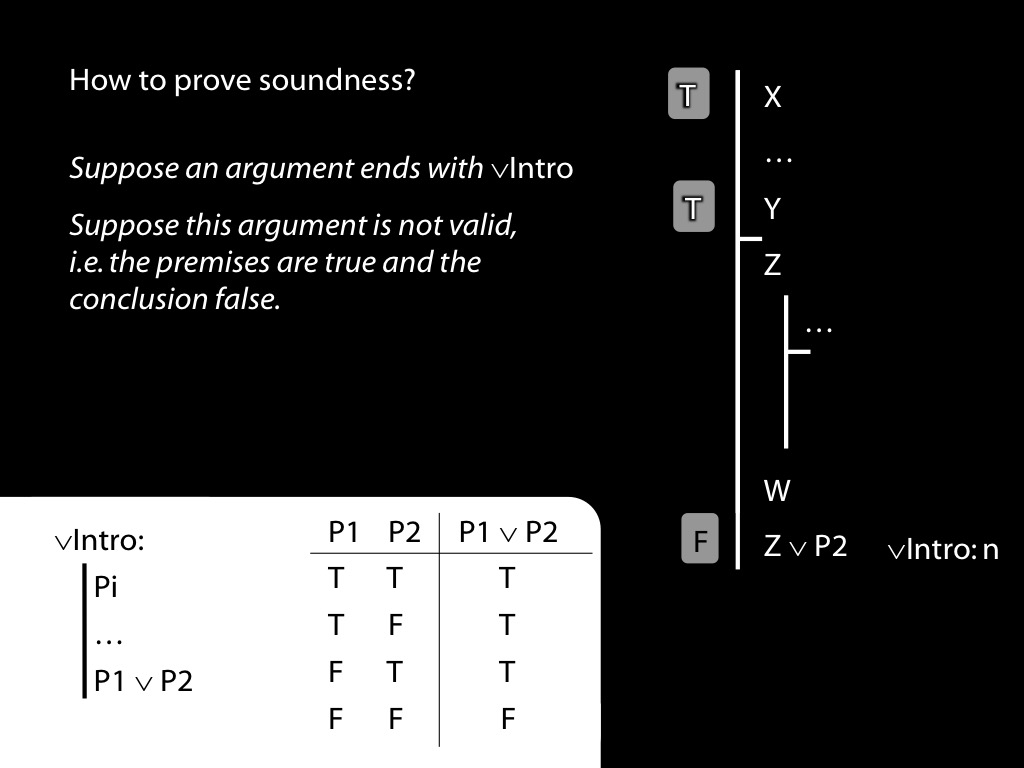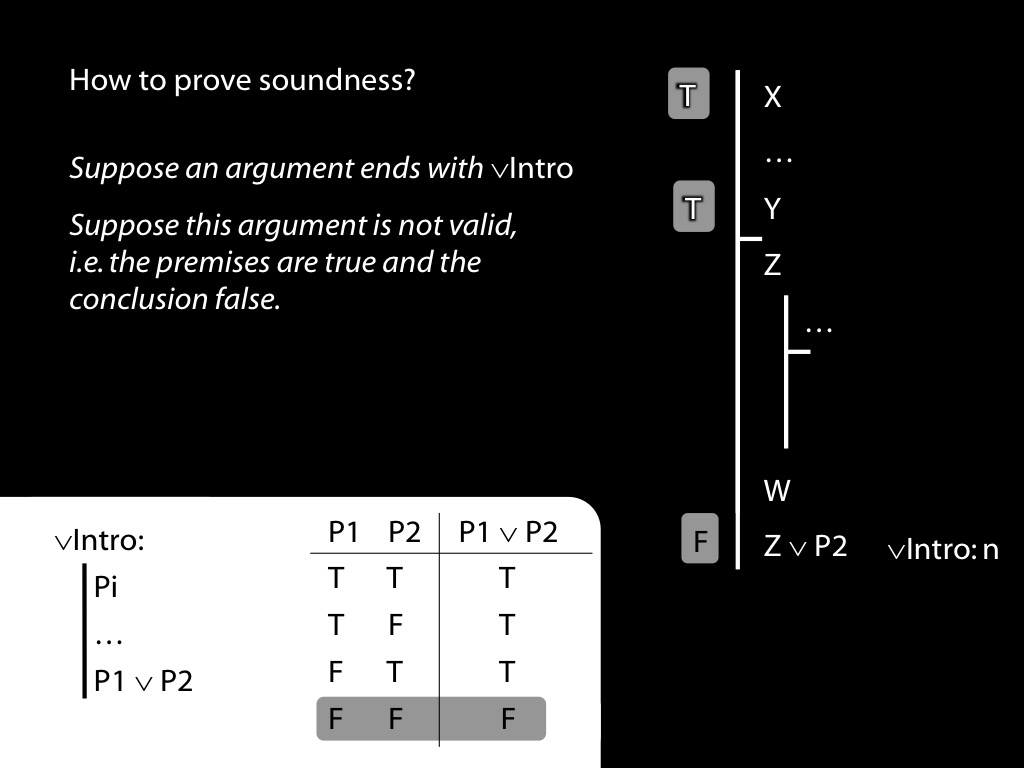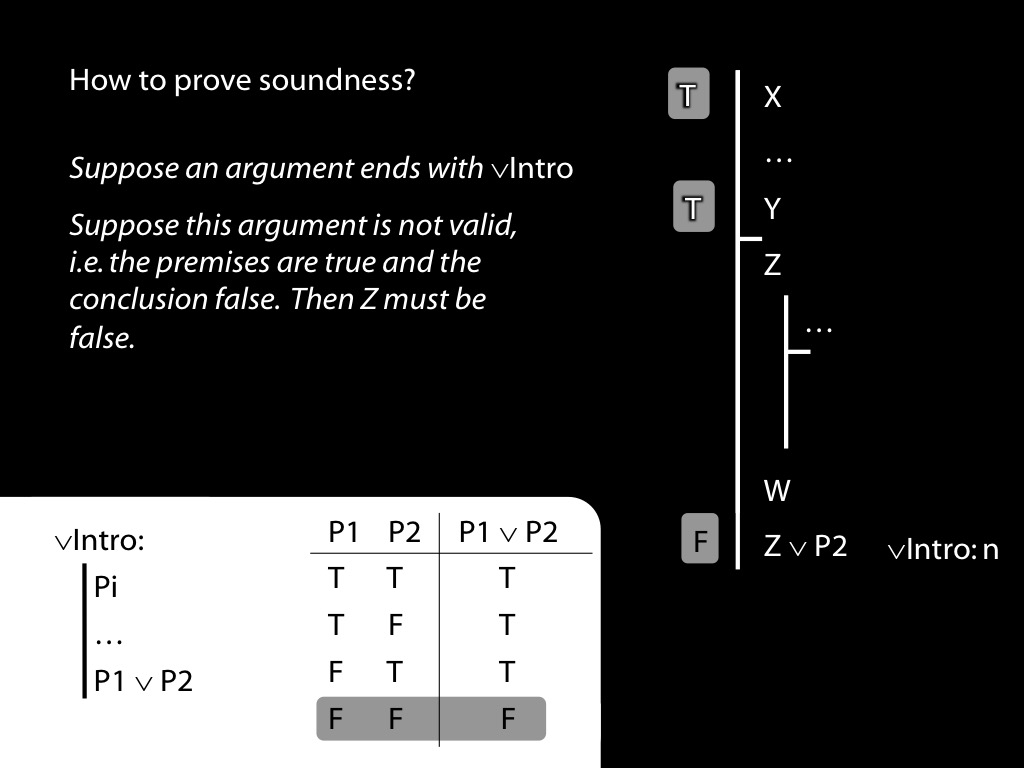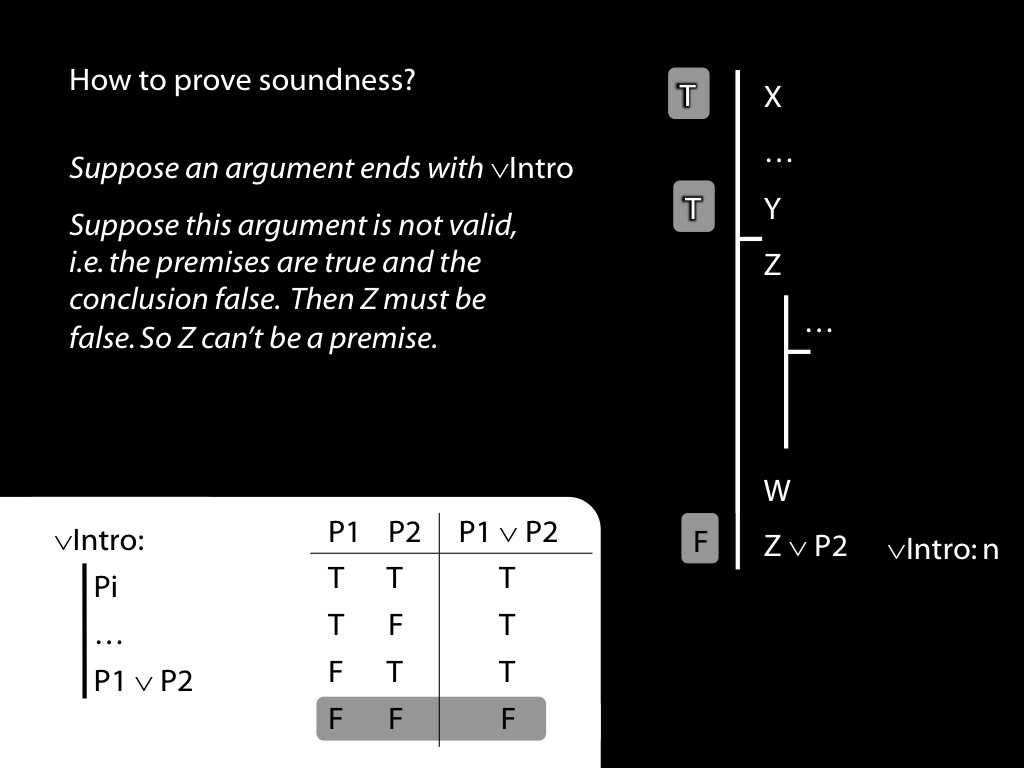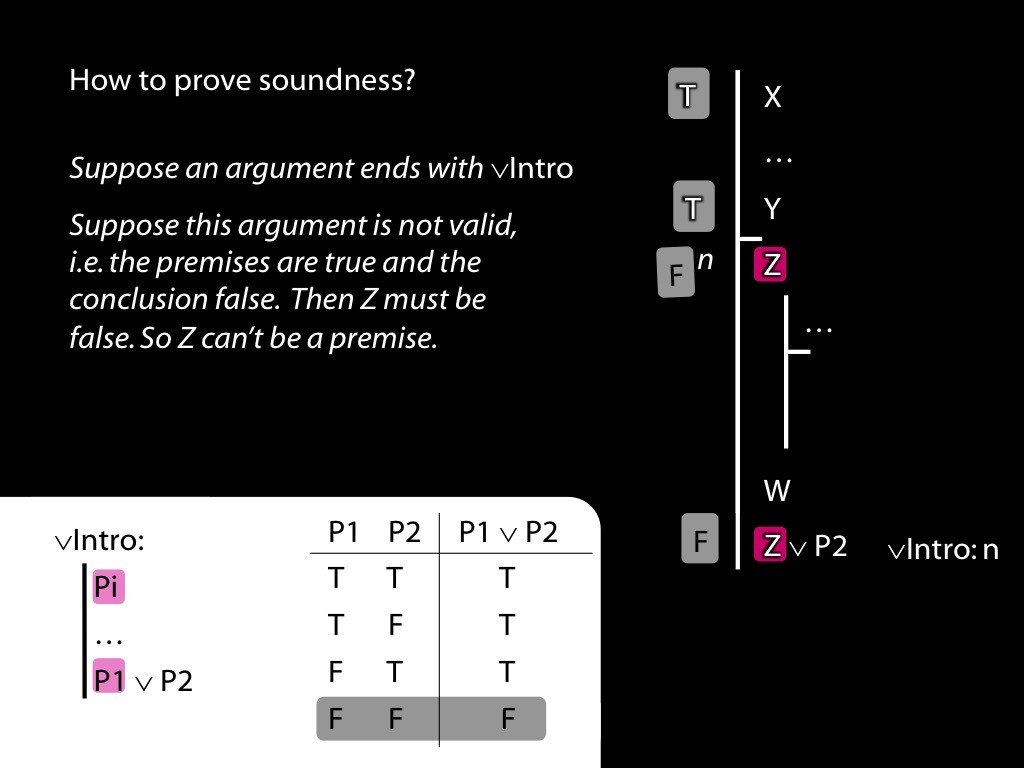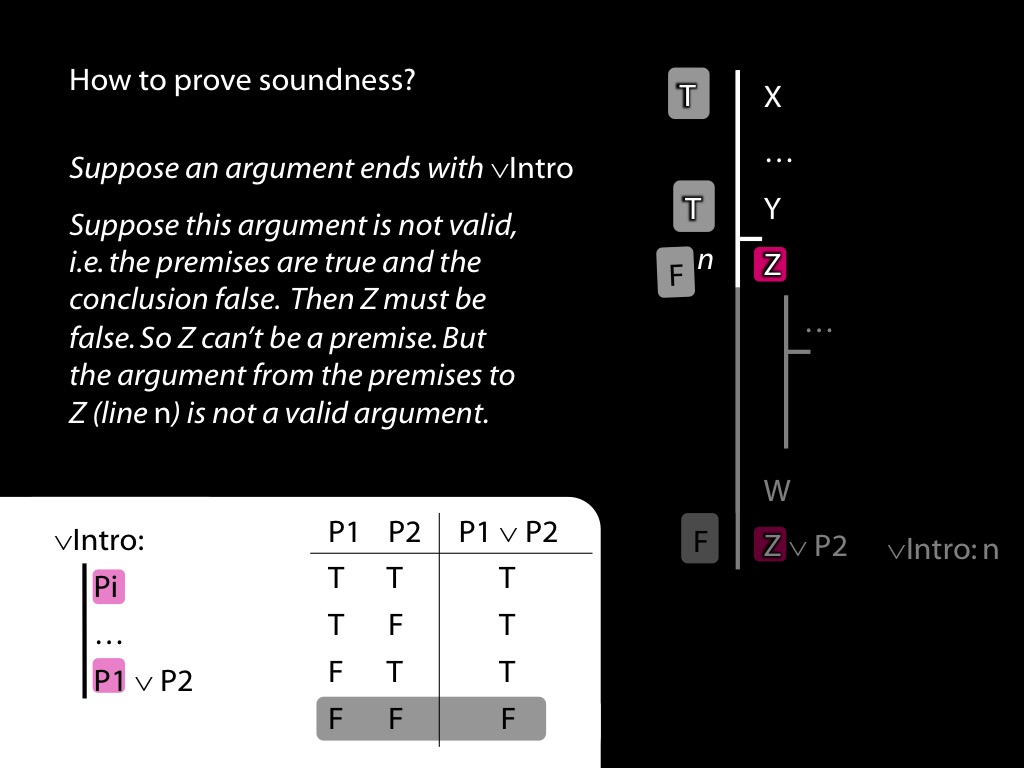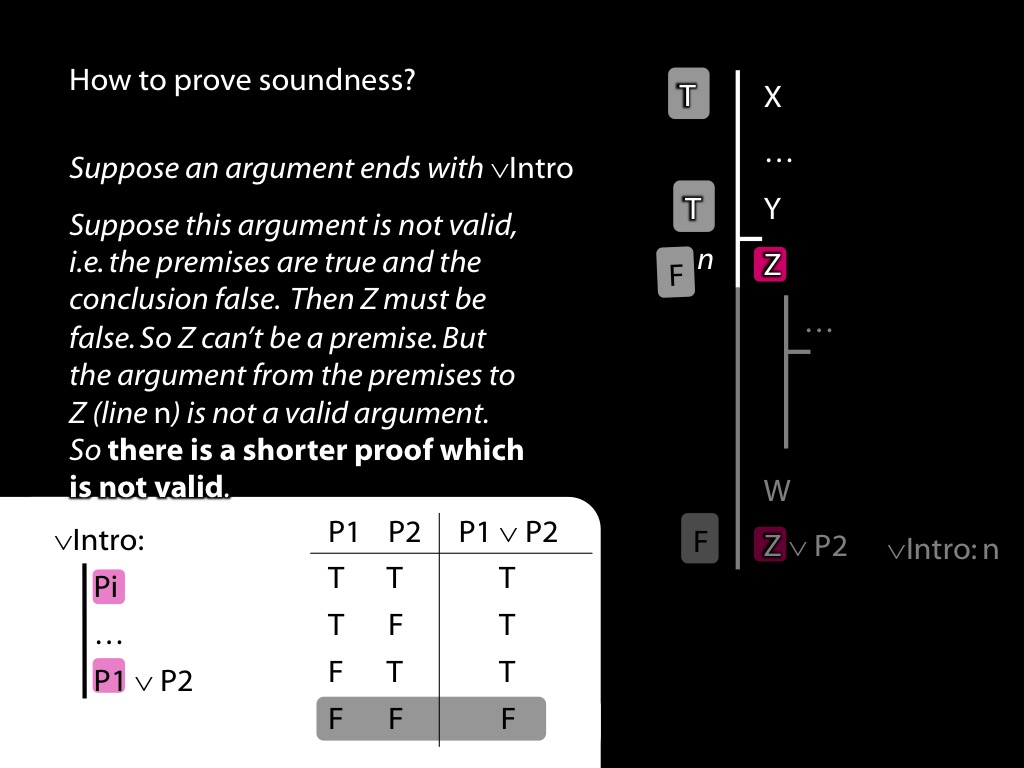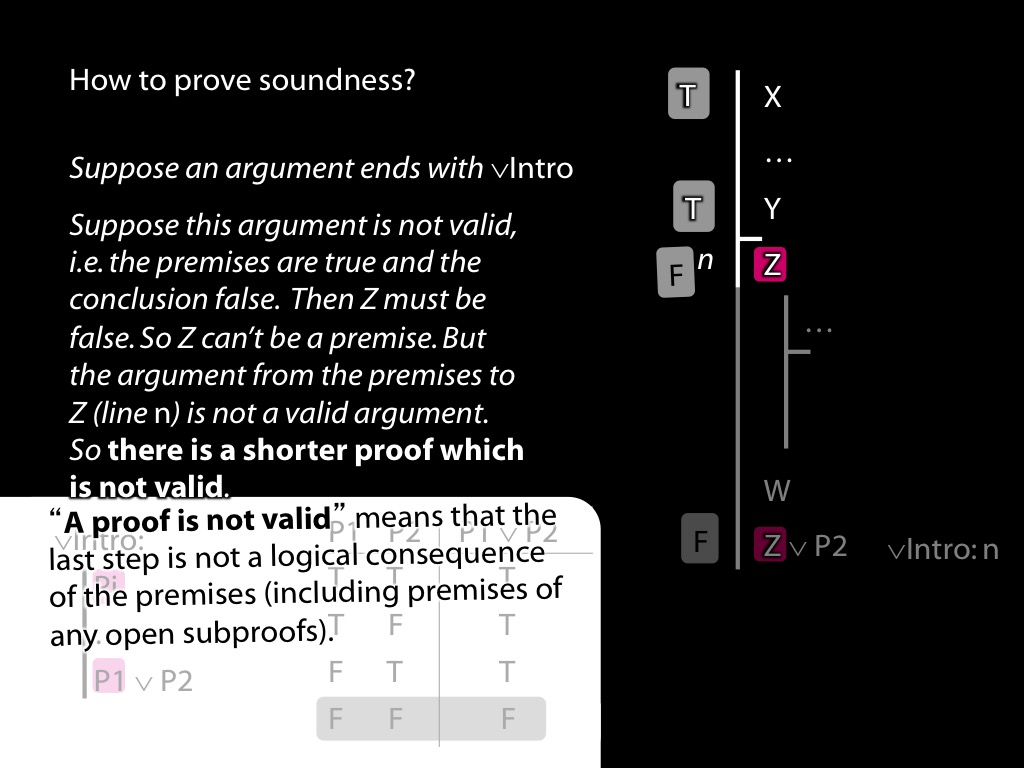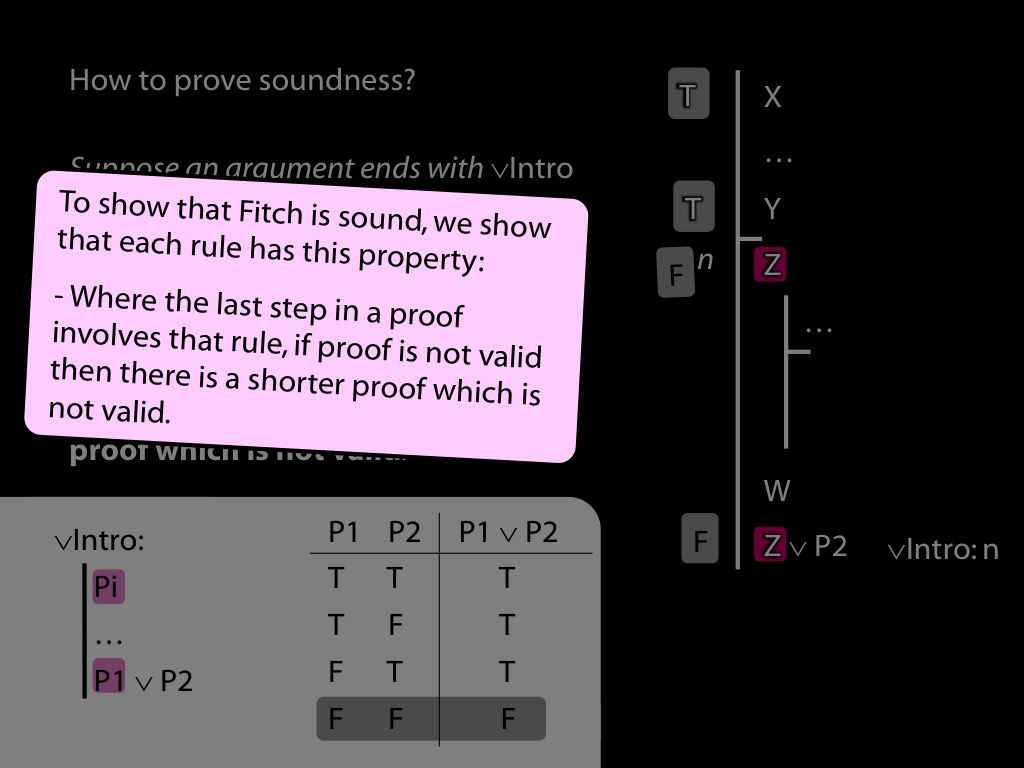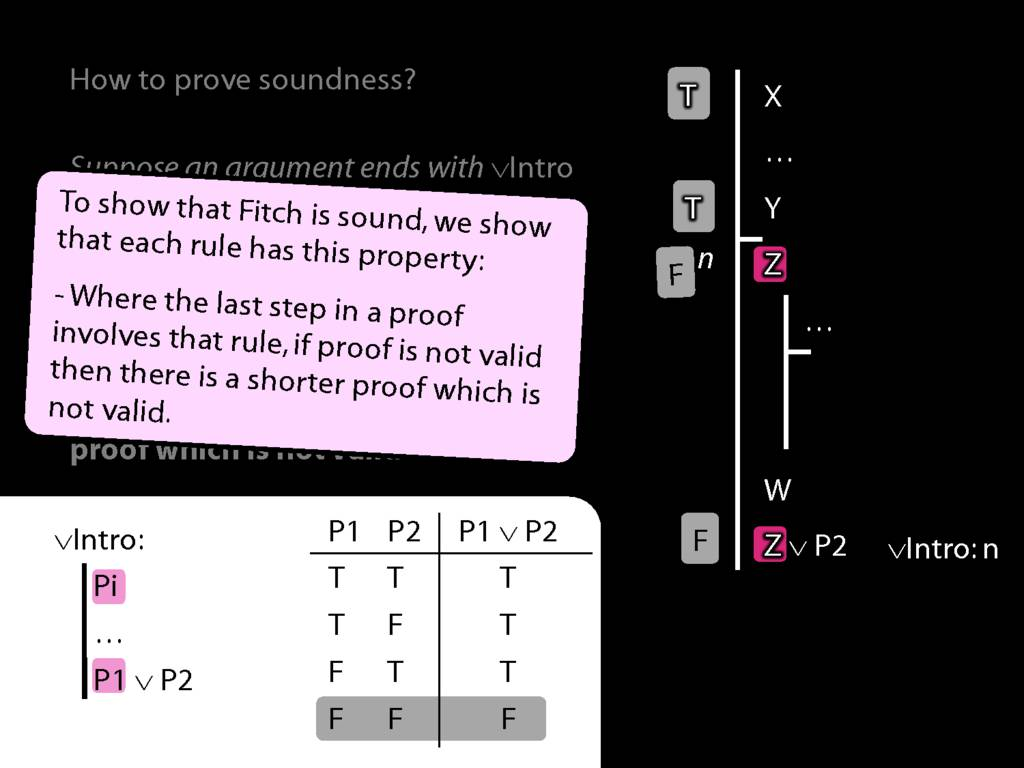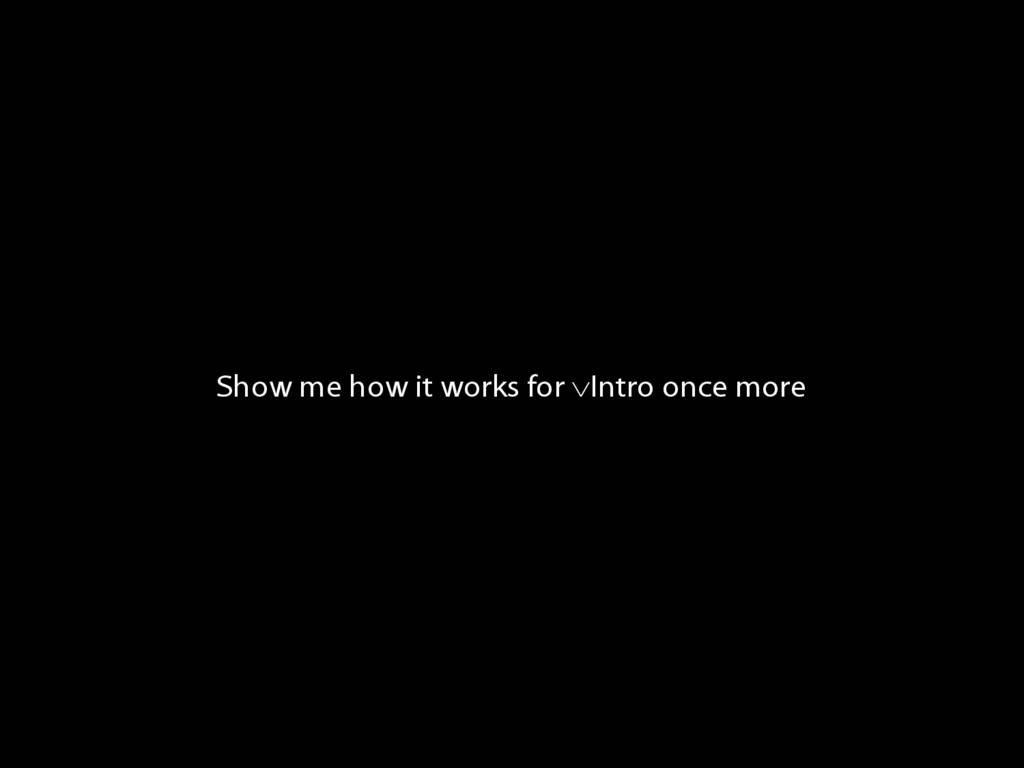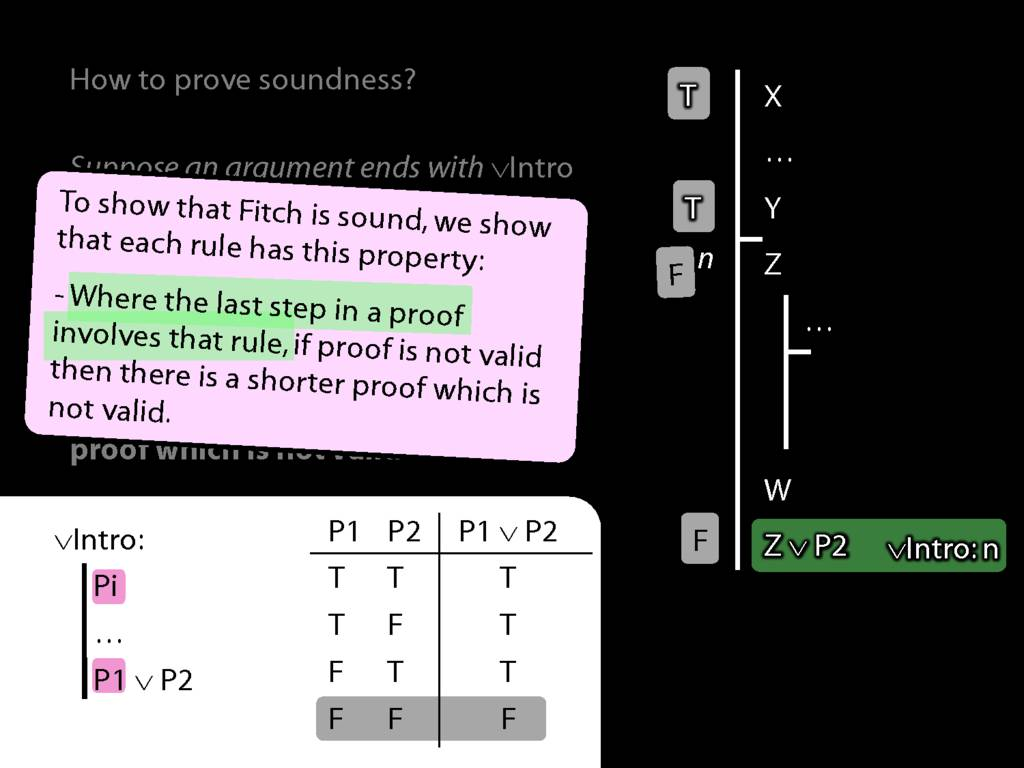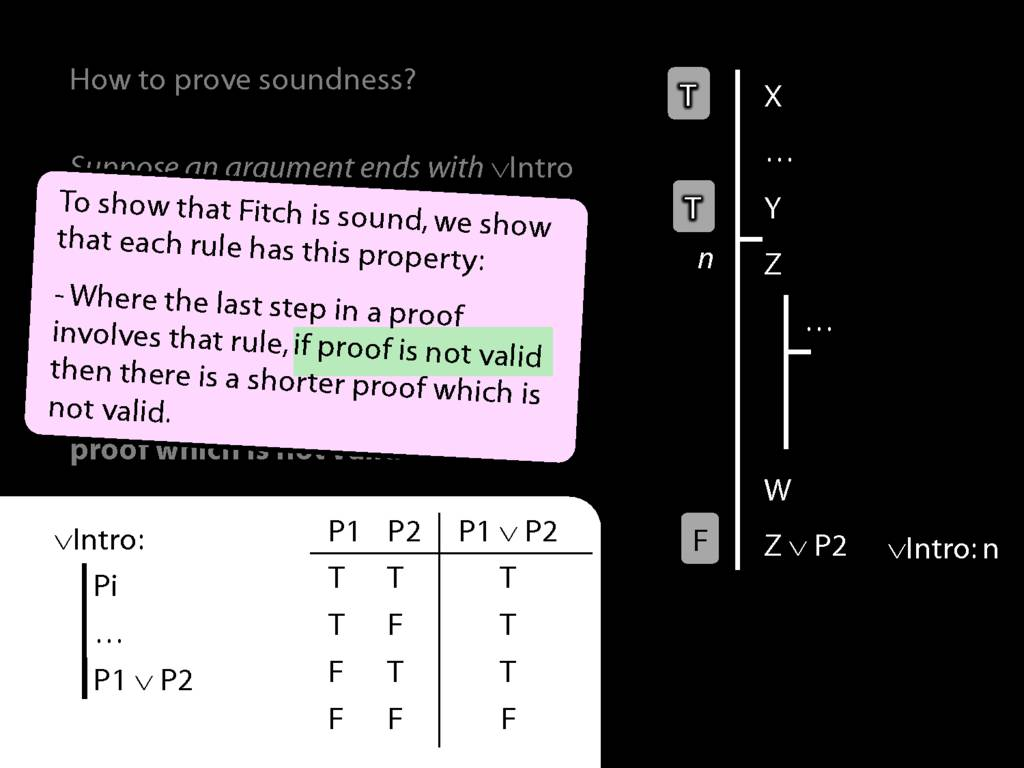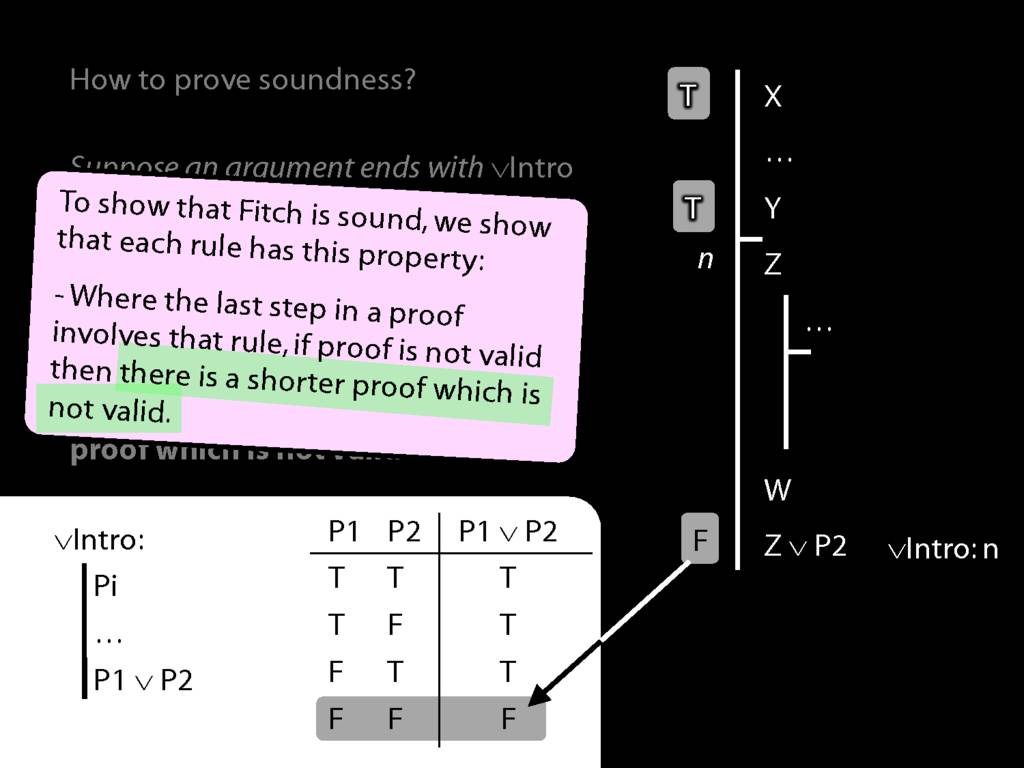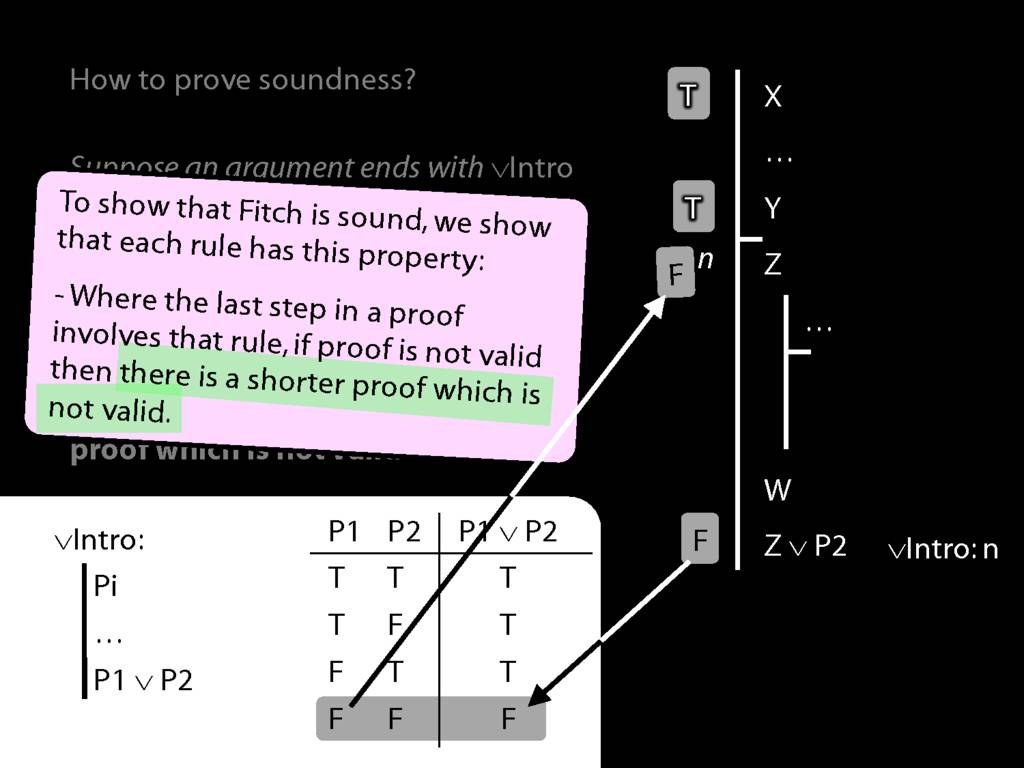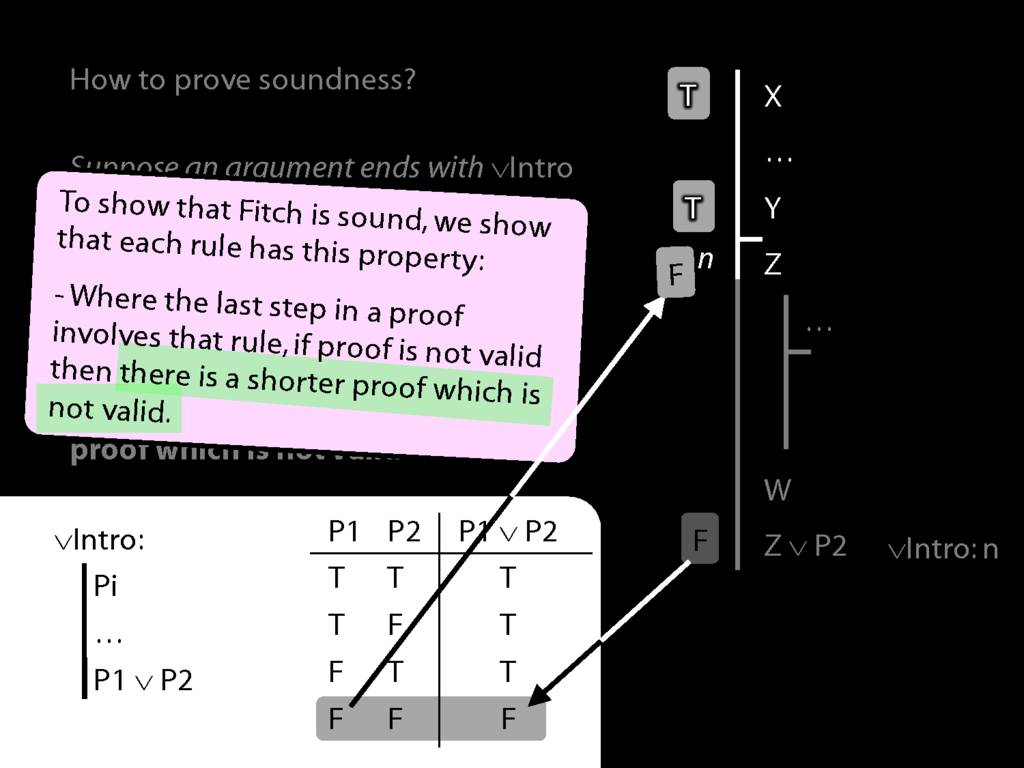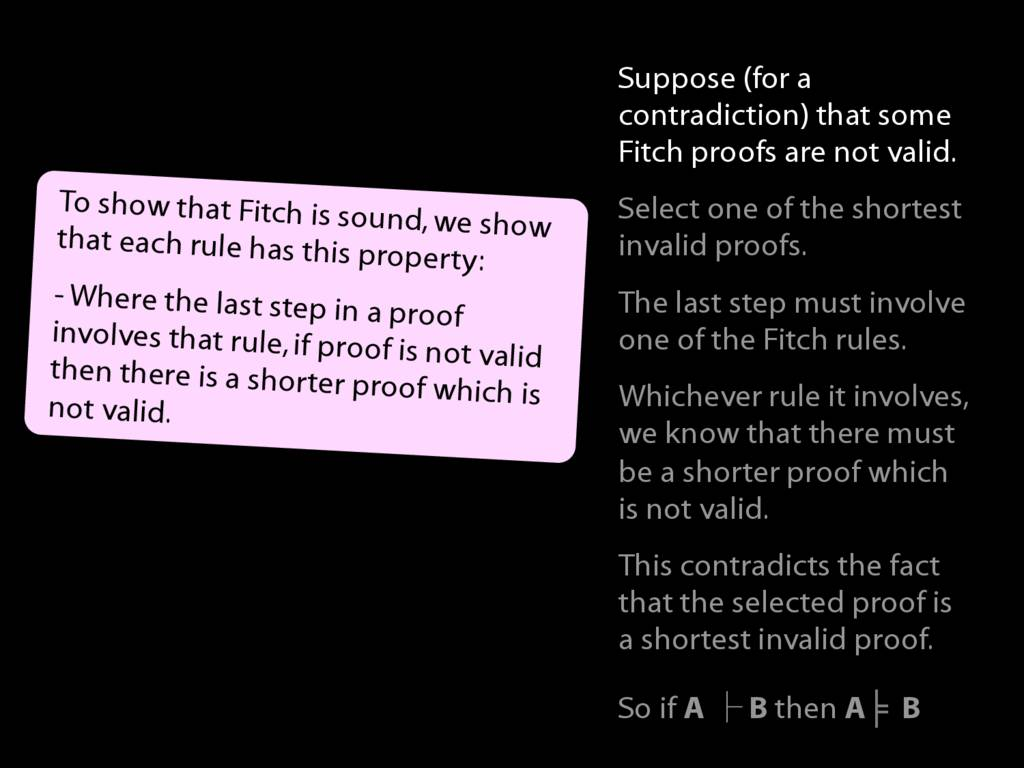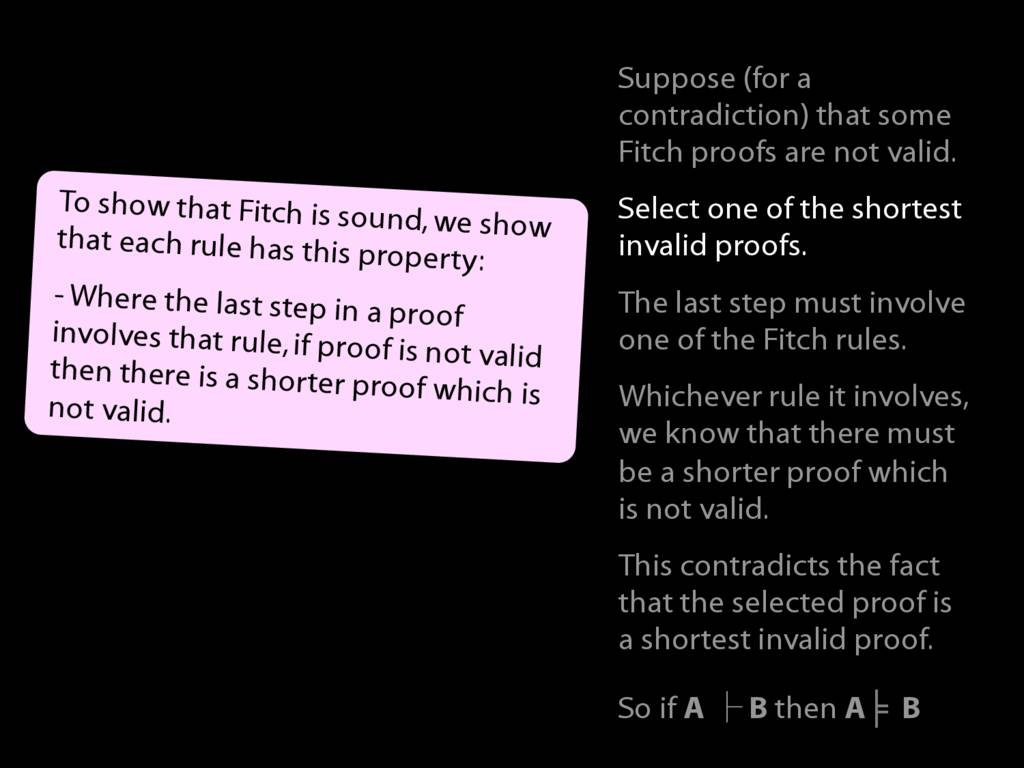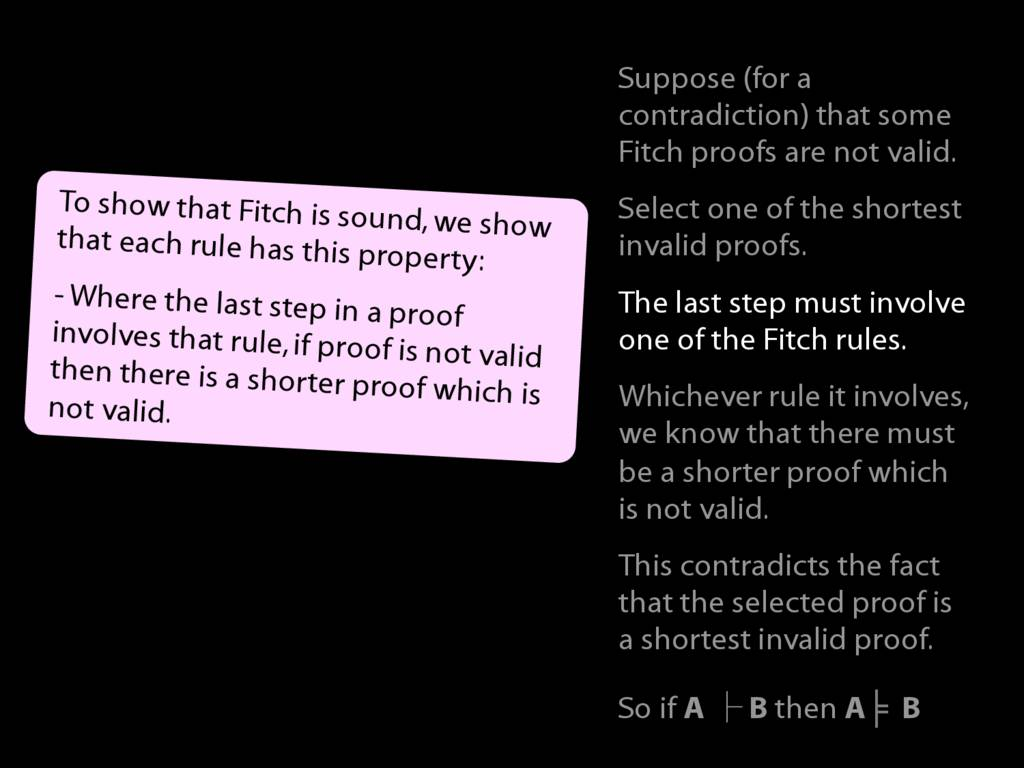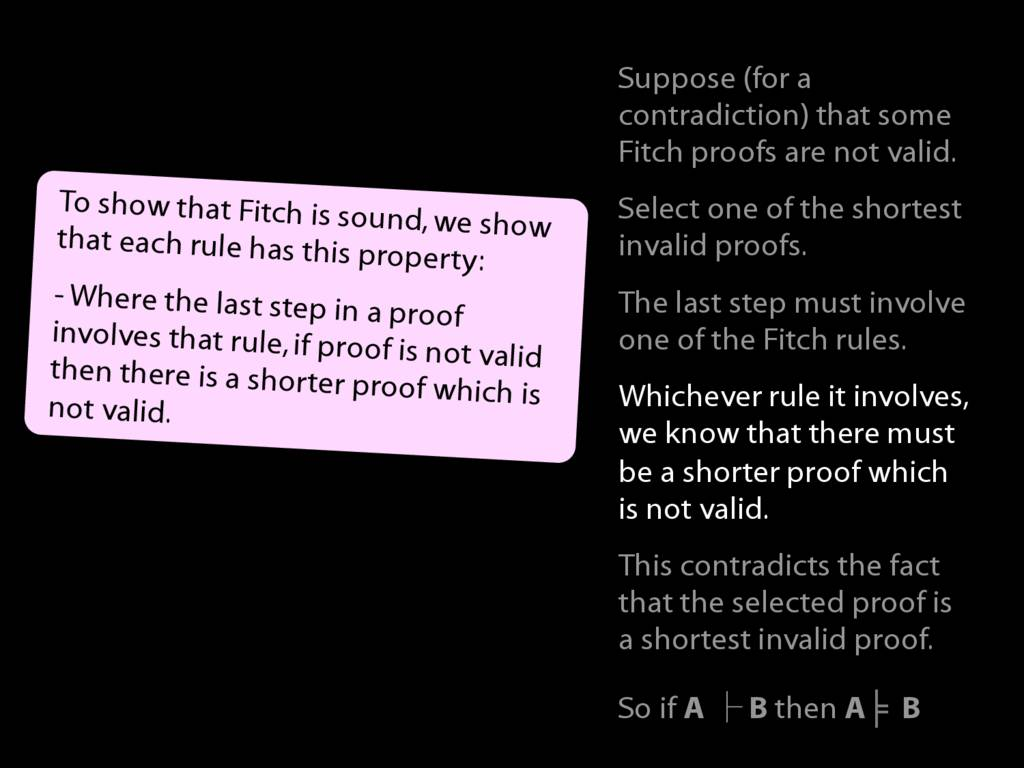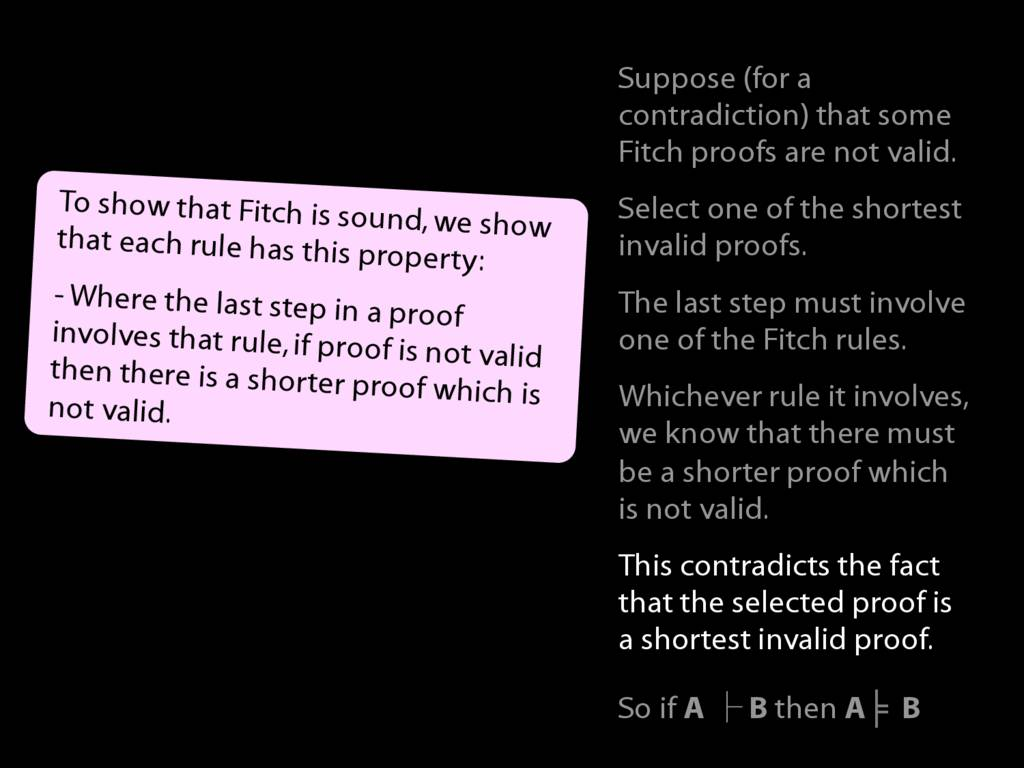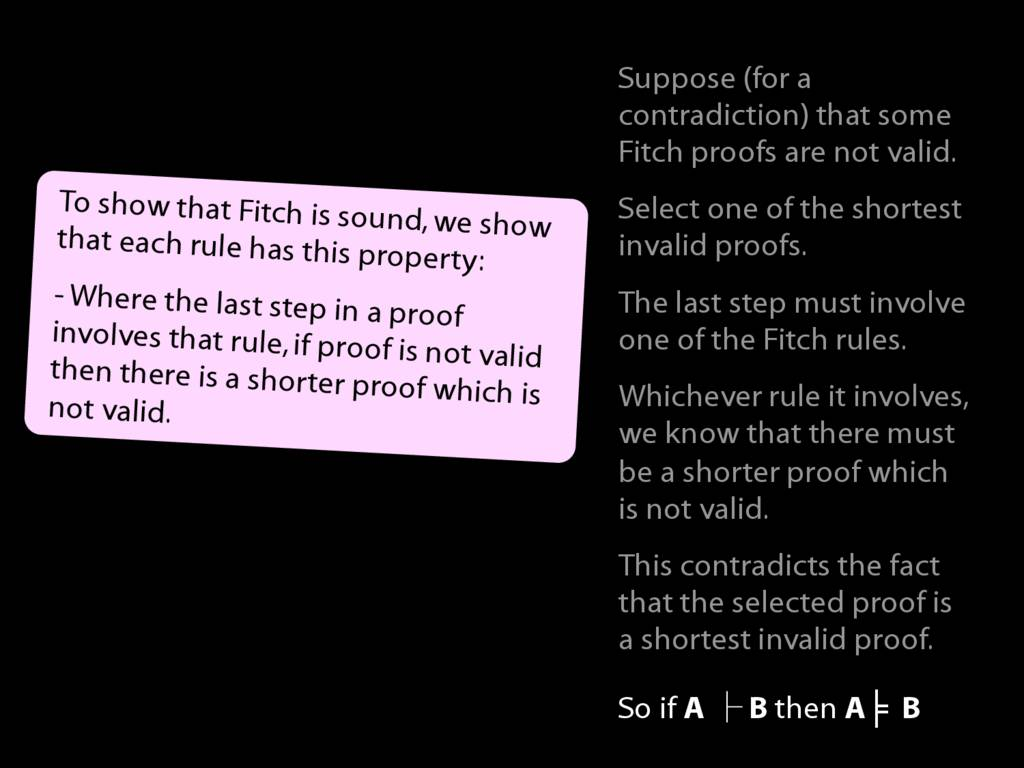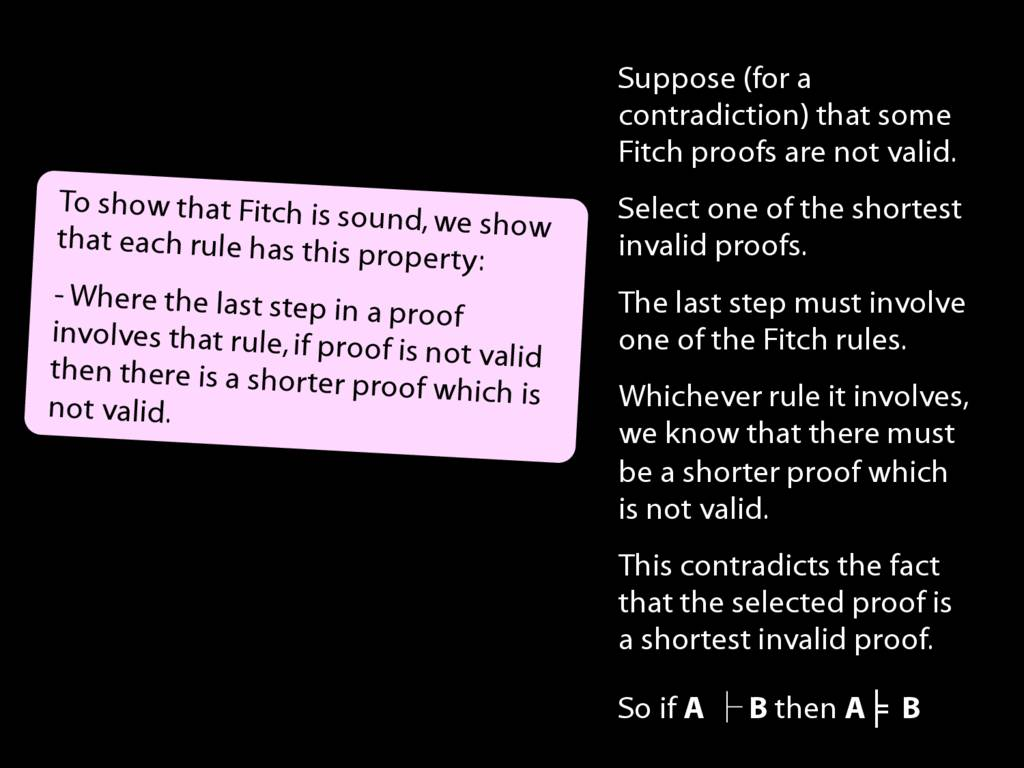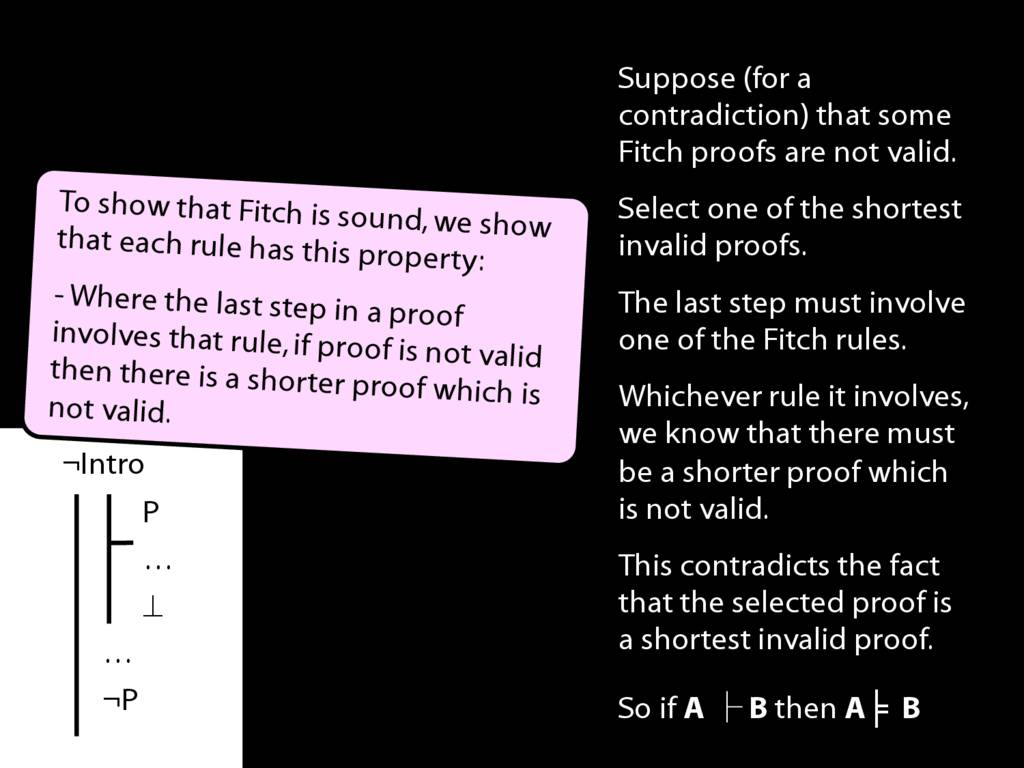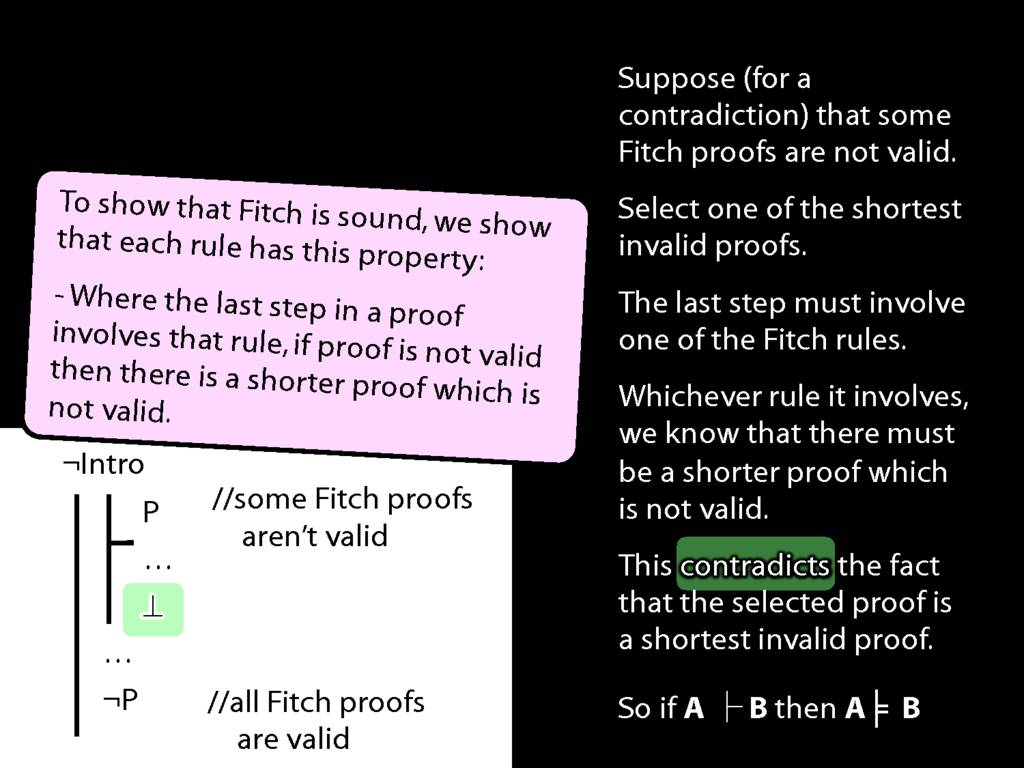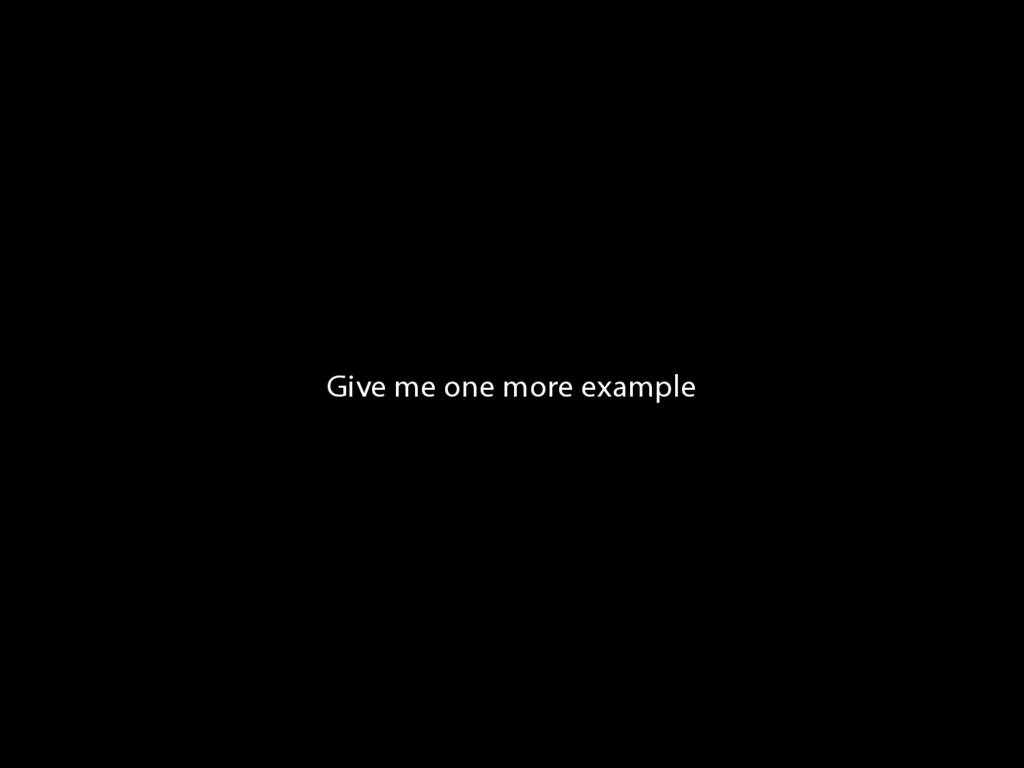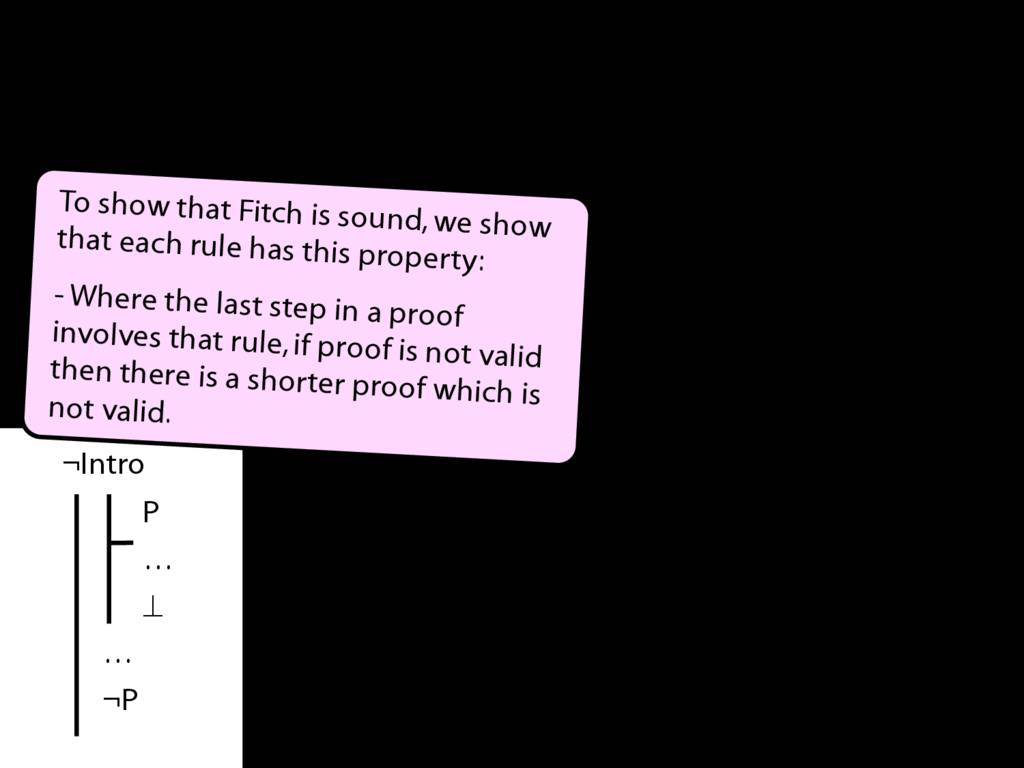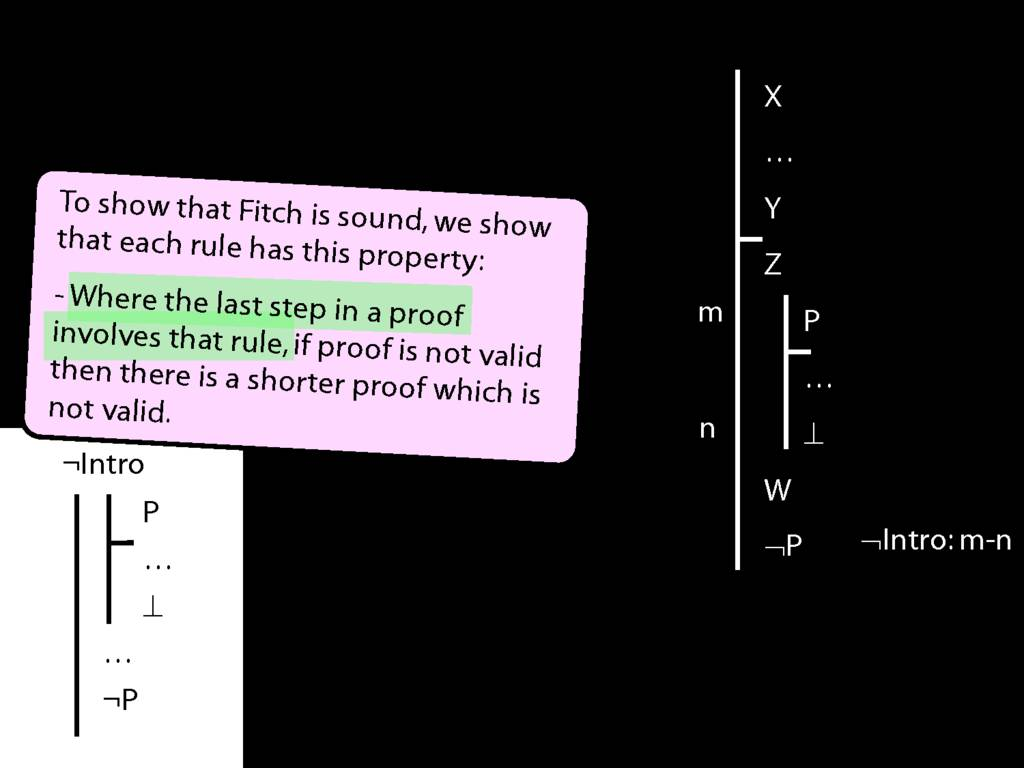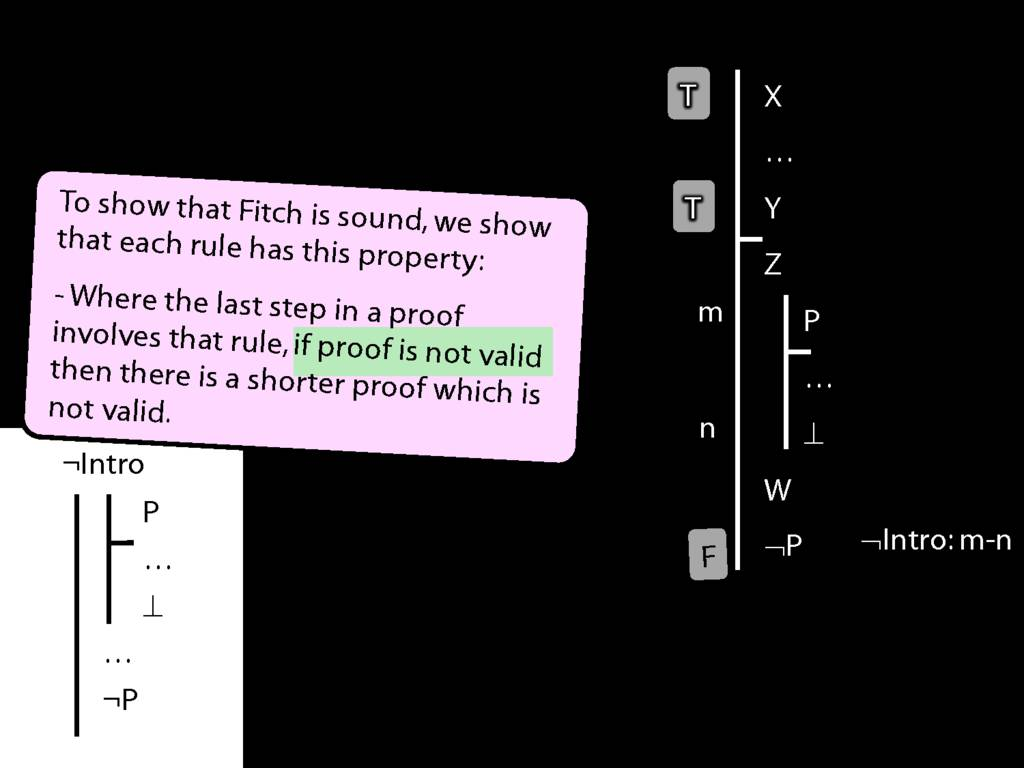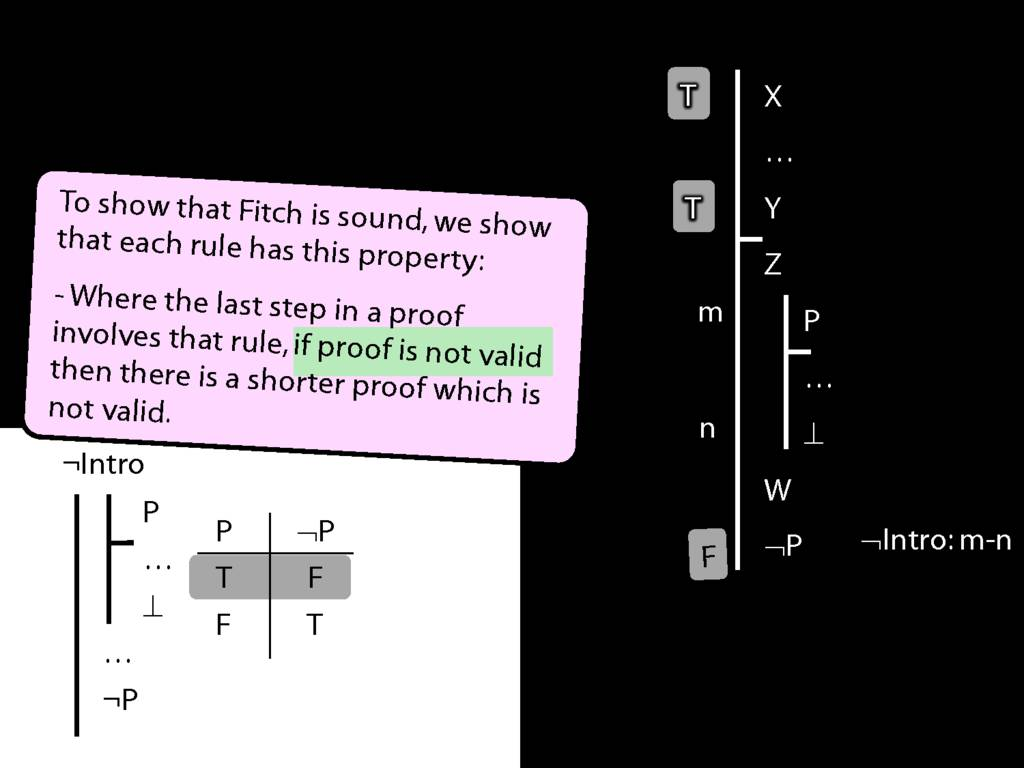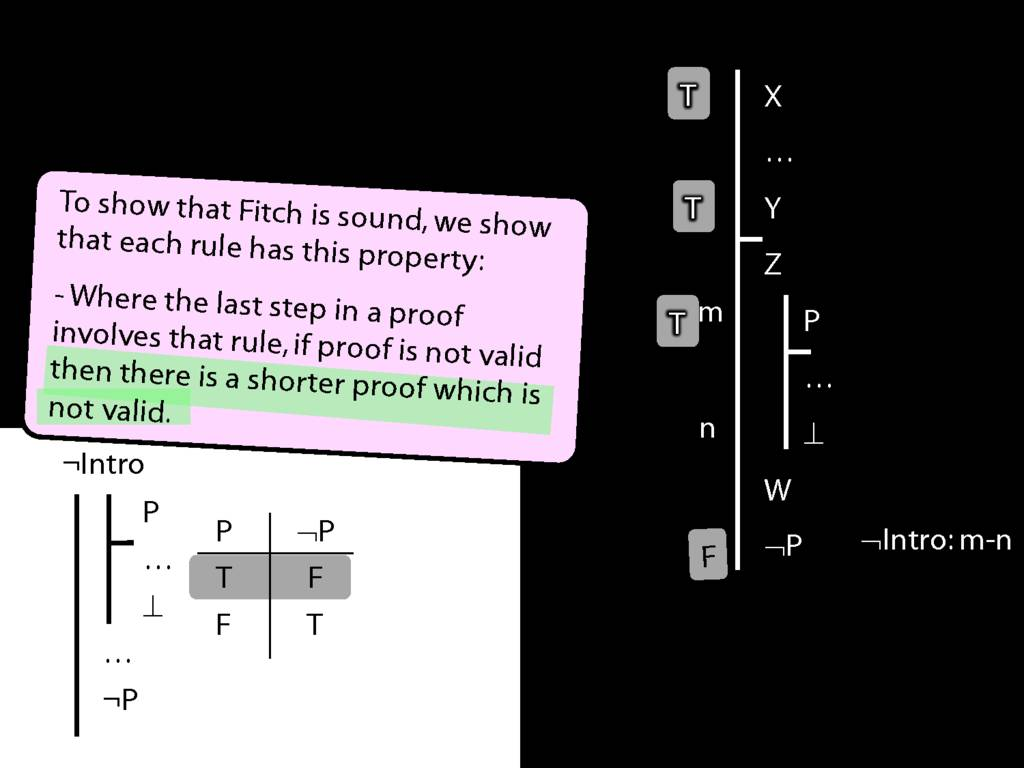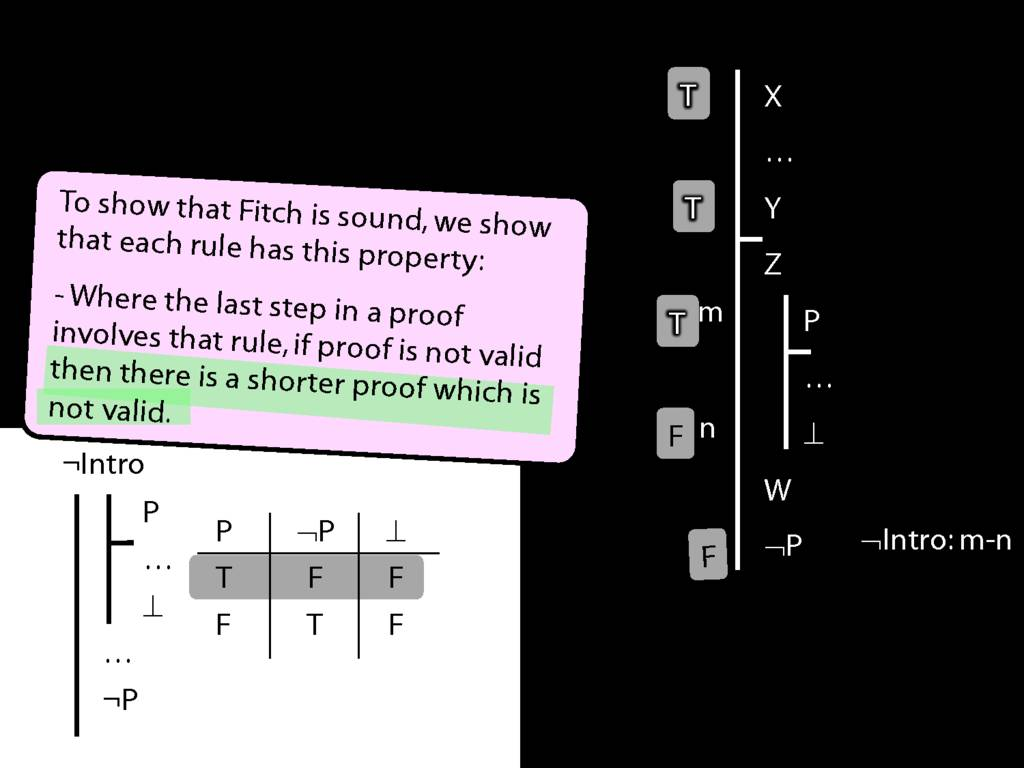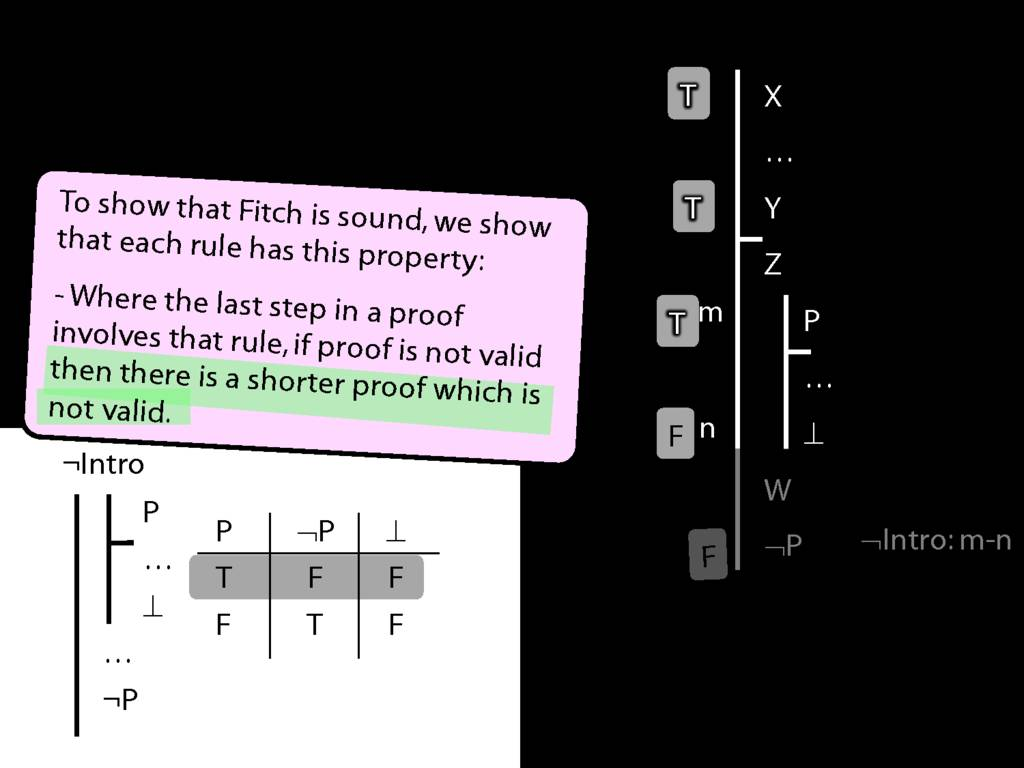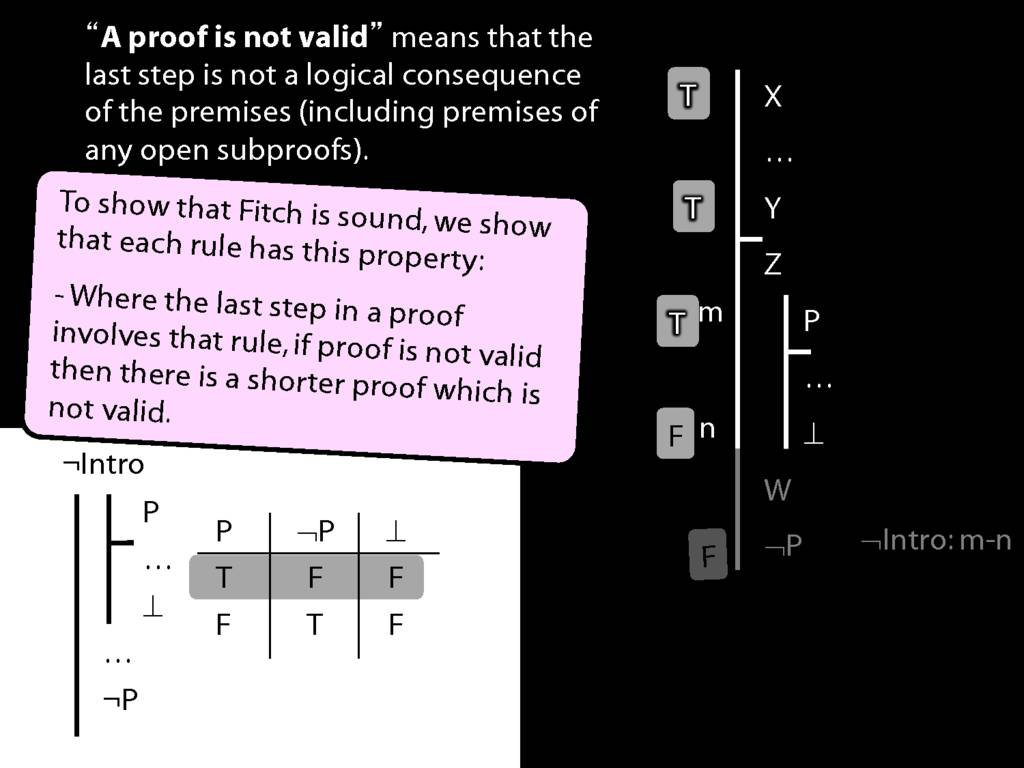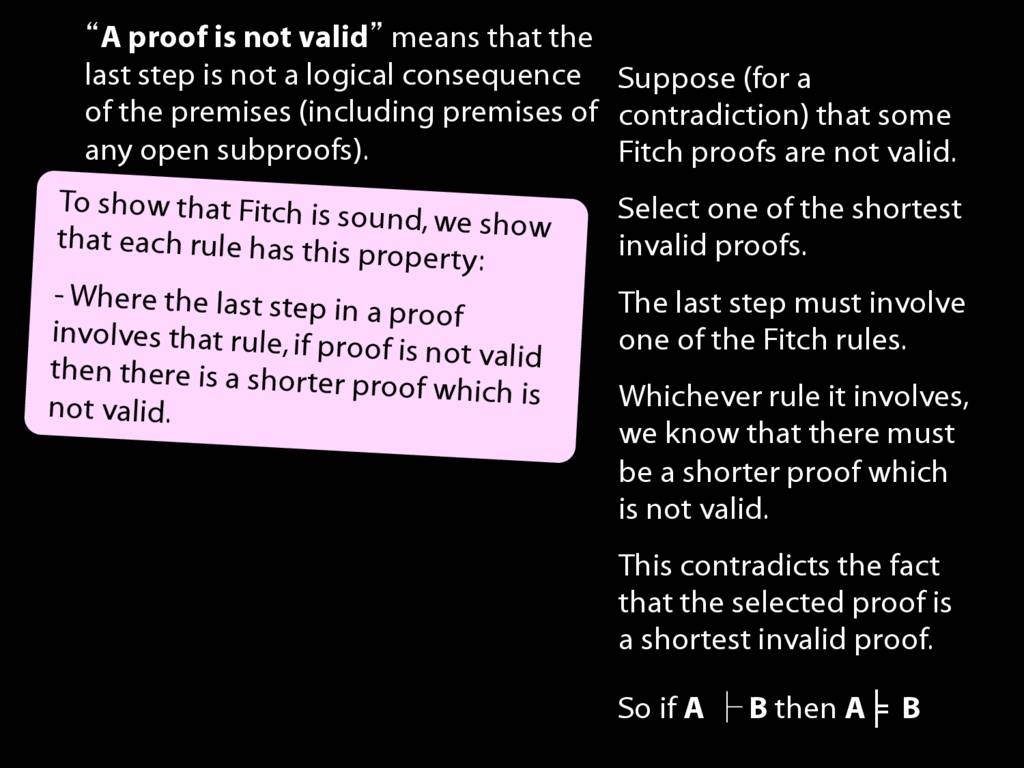Press the right key for the next slide (or swipe left)

also ...

Press the left key to go backwards (or swipe right)

Press n to toggle whether notes are shown (no equivalent if you don't have a keyboard)

Press m or double tap to see a menu of slides

## Proof of the Soundness Theorem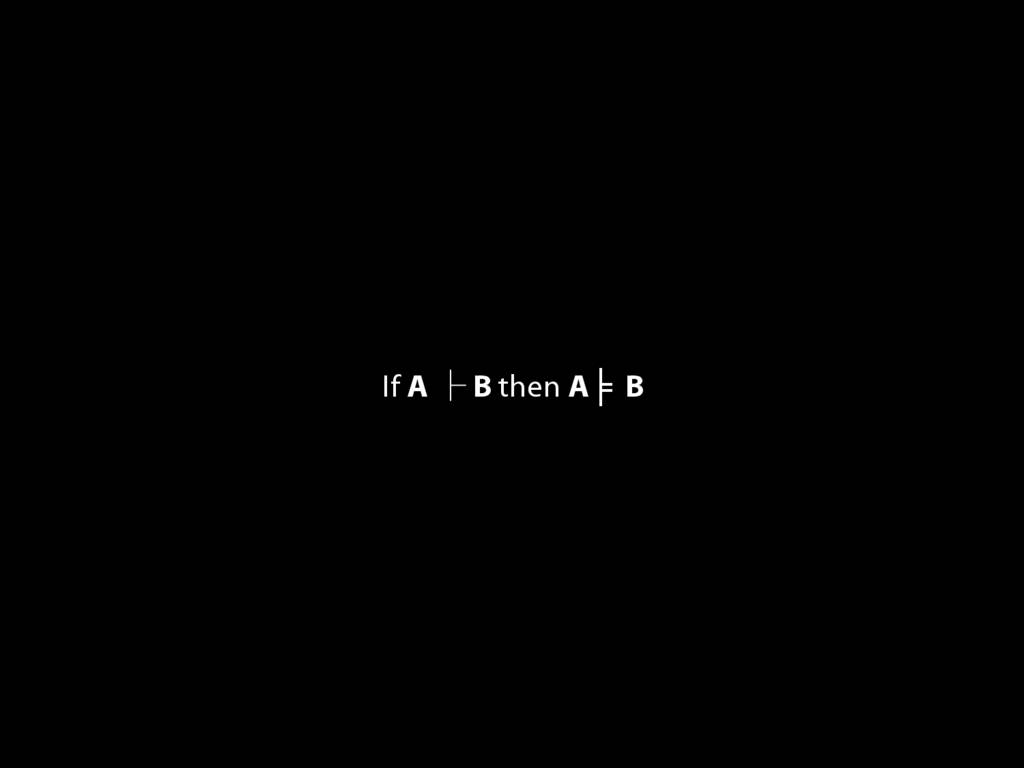\begin{minipage}{\columnwidth}
\textbf{Illustration of soundness proof: ∨Intro}
\end{minipage}
\emph{Useful Observation about any argument that ends with ∨Intro.} Suppose this argument is not valid, i.e. the premises are true and the conclusion false. Then Z must be false. So the argument from the premises to Z (line n) is not a valid argument. So there is a shorter proof which is not valid.
\emph{Stipulation}: when I say that \emph{a proof is not valid}, I mean that the last step of the proof is not a logical consequence of the premises (including premises of any open subproofs).
\begin{minipage}{\columnwidth}
\textbf{Illustration of soundness proof: ¬Intro}
\end{minipage}
\begin{minipage}{\columnwidth}
\textbf{How to prove soundness? Outline}
Step 1: show that each rule has this property:
\hspace{5mm} Where the last step in a proof involves that rule, if proof is not valid then there is a shorter proof which is not valid.
Step 2: Suppose (for a contradiction) that some Fitch proofs are not valid. Select one of the shortest invalid proofs. The last step must involve one of the Fitch rules. Whichever rule it involves, we know that there must be a shorter proof which is not valid. This contradicts the fact that the selected proof is a shortest invalid proof.
\end{minipage}